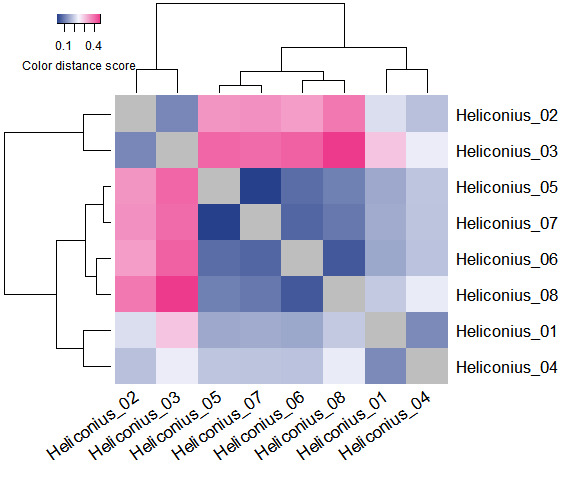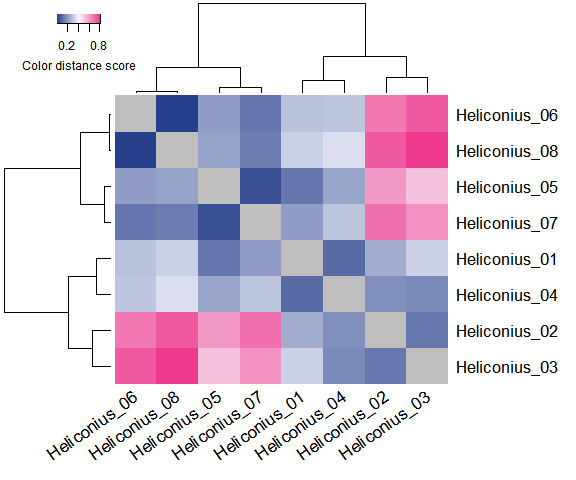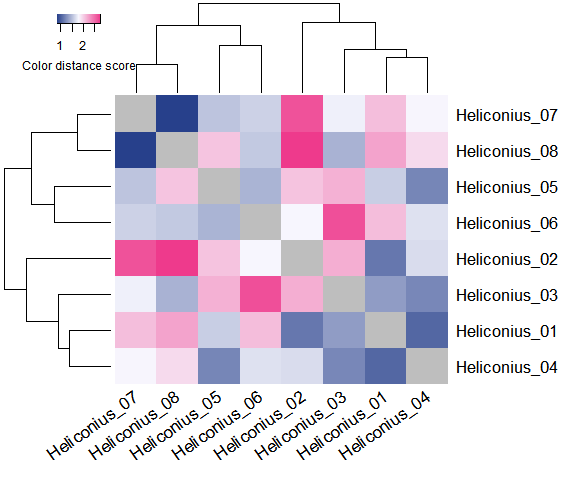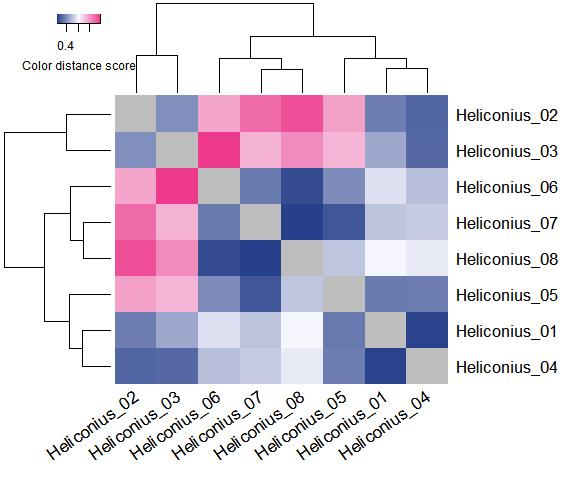# Color Distance Metrics

## Introduction

The last step in calculating a color distance matrix for a set of images is to choose a method for measuring the distance between sets of color clusters, whether they were computed using color histograms or k-means clustering. The clusters summarize two important pieces of information about an object: the colors present in the image, and the relative proportion of each color in the image. To measure the color similarity of two objects, a distance metric should take both of these features into account.

colordistance provides four metrics for quantifying the similarity of binned images, which emphasize different features of the dataset. All of them are implemented by specifying the method argument of getColorDistanceMatrix(), which takes a list of cluster sets as returned by getHistList() or extractClusters() (see binning methods), and they implemented in essentially the same way. For every unique combination of two different images in a set, the distance metric is calculated between the cluster sets of those two images, and that value is stored in a symmetrical distance matrix which is returned as a matrix object with row and column names taken from the original image names. The methods are described below, along with some advice on choosing an appropriate distance metric for a dataset.

## Earth Mover’s Distance

Specified with method="emd" in getColorDistanceMatrix().

The earth mover’s distance or Wasserstein metric measures the distance between two distributions as a transport cost – essentially, what is the minimum cost of transforming one distribution into the other? It takes into account both spatial color information (where a cluster is in 3D color space) and size information (how large a cluster is, i.e. how much earth you have to shovel), using the emd() function from the emdist package to compare 3D histograms. In part, it implements the Hungarian algorithm to match up bins that are most similar both in color and size, which tends to mitigate the effect of dividing pixels up into arbitrary ranges.

EMD is the default for most colordistance functions, and typically performs well because it accounts for all of the information in the histograms and allows for partial matches in a quantitative, scaleable way. So even if one color histogram has a large grey bin and no pixels in the black bin where another one has a large black bin and an empty grey one, the similarity of black and grey result in a lower transport cost than if the bins were treated as independent.

However, if you care more about color presence/absence than amount, or care only about the distributions of certain colors, etc – if you don’t want to take all of the available color information into account – this metric may not be ideal.

## $$\chi^{2}$$ Distance

Specified with method="chisq" in getColorDistanceMatrix().

Beyond the color similarity guaranteed by using the same set of bins with histogram binning or by setting ordering=TRUE for extractClusters() with k-means, $$\chi^{2}$$ distance doesn’t take color distance information into account at all, but instead returns the sum of the $$\chi^{2}$$ distances for every pair of bins. For example, the distance between images $$a$$ and $$b$$ is calculated as follows, where $$n$$ is the number of bins:

$\sum_{i=1}^{n} \frac{(a_i - b_i)^{2}}{(a_i + b_i)}$

Bins are treated as independent – bin 1 in image 1 is compared to bin 1 in image 2, and so on. If bin 1 in image 1 is very large and bin 1 in image 2 is empty, the distance will increase dramatically, even if bin 2 in image 2 is very large and similar in color to bin 1 in image 1. So a black object would look as different from a grey object as it might from a bright yellow one, even though you would intuitively rank the black and grey objects as being more similar to each other.

In practice, despite being fairly crude, $$\chi^{2}$$ distance actually performs about as well as EMD in many cases. Color histograms guarantee that the pixels grouped into specific bins will at least be fairly similar in color across different images since they share the same bounds. The only cases where it performs noticeably poorly are when colors or color families fall along the boundaries of bins so they are treated separately, or if k-means clustering was used for binning, since that method tends to divide up dominant colors into different clusters of variable size.

## Color Distance

Specified with method="color.dist" in getColorDistanceMatrix().

The color distance metric calculates the Euclidean distance in color space between each pair of clusters, ignoring their size. The distance between images $$a$$ and $$b$$ (using RGB) is calculated as follows, where $$n$$ is the number of bins:

$\sum_{i=1}^{n} \sqrt((R^{a}_{i}-R^{b}_{i})^{2} + (B^{a}_{i}-B^{b}_{i})^{2} + (G^{a}_{i}-G^{b}_{i})^{2})$

It’s essentially the opposite of the $$\chi^{2}$$ metric above, which ignores color information and takes into account only the size differences of clusters in the same region – color distance emphasizes differences in the locations of the clusters, but not their sizes.

In order for this metric to have any meaning, it is necessary to compare the most similar colors in images to each other. So unordered k-means clusters (using ordering=FAlSE for extractClusters()) would not produce useful results with this metric, since bin 1 in image 1 may be yellow while bin 1 in image 2 is pink, even though both images contain both yellow and pink clusters, etc; this would artificially inflate the distance sum and make the images look more similar than they are.

On the other hand, if you use histogram binning and use the bin centers for the cluster values (if bin.avg=FALSE for getImageHist() or getHistList()), you’ll always end up with a distance of 0 between images, because the cluster centers are always going to have the same coordinates.

Color distance is generally appropriate only if you specifically want to ignore size information. But in many cases, specifically with dominant colors, natural color clusters will still get broken up into several different clusters – a mostly yellow image might have yellow pixels present in 3 different clusters, for example. Using color distance would then compare each of the three yellow clusters against individual clusters from another image, so yellow would be overrepresented, and so on. That said, color distance will downplay the cluster size much more than the other methods.

## Weighted Pairs Distance

Specified with method="weighted.pairs" in getColorDistanceMatrix().

Weighted pairs is similar to earth mover’s distance in that it accounts for both color and size, but it takes optional user weights for the color and size components. Essentially, it calculates two distance matrices: one using $$\chi^{2}$$ distance for sizes, and one using color distance for cluster centers. It then combines them according to the specified weights.

If ordering=TRUE, the bins are matched in such a way that the overall sum of size distances + color distances for all bin pairs is minimized. Otherwise, bins are paired by the order in which they’re given. Like EMD, the reordering is accomplished by an implementation of the Hungarian algorithm.

The number of moving parts in this distance metric – ordering, size weight, color weight, and the specifics of how the bins were calculated in the first place – make weighted pairs distance a little dicey. Unless you have a good reason for needing to precisely tweak the relative weights of cluster sizes and colors in an analysis, EMD will usually give better results.

## Choosing a distance metric

The metrics above are listed in order of recommendation – EMD will do the best job for most analyses; $$\chi^{2}$$ does well as long as the bins are paired up appropriately (so color histograms or if ordering=TRUE for k-means); color distance and weighted pairs will only be useful in specialized cases. That said, it’s easy enough to try them all out by just altering the method argument of getColorDistanceMatrix() or the distanceMethod argument of imageClusterPipeline() and inspecting the results.

Unlike the binning methods, all of the distance metrics take approximately the same amount of time – choosing one over the other shouldn’t dramatically impact the calculation time for a distance matrix once the clusters have been calculated. For larger datasets (either many images or many bins), however, $$\chi^{2}$$ will almost invariably be fastest because it ignores spatial color information, performs fewer calculations, and those calculations it does perform are one-dimensional. There may therefore be cases where it does equally as well as EMD, but much faster. Otherwise, start off using EMD, and if it’s not returning useful results, test out the other metrics.

## Cheatsheet

images <- dir(system.file("extdata", "Heliconius/", package="colordistance"), full.names=TRUE)

# First get the cluster sets
clusters <- colordistance::getHistList(images, lower=rep(0.8, 3), upper=rep(1, 3))
## Warning in colordistance::getHistList(images, lower = rep(0.8, 3), upper = rep(1, : RGB and HSV are device-dependent, perceptually non-uniform color spaces. See 'Color spaces' vignette for more information.
## Using 3^3 = 27 total bins
# Distance metrics -- note that each one produces a different set of clusters, but some are more similar than others

# Using earth mover's distance
EMD_CDM <- colordistance::getColorDistanceMatrix(clusters, method="emd")# Using chi-squared distance
chisq_CDM <- colordistance::getColorDistanceMatrix(clusters, method="chisq")# Using color distance
color_CDM <- colordistance::getColorDistanceMatrix(clusters, method="color.dist")# Using weighted pairs with uneven weights and ordering off
weighted_CDM <- colordistance::getColorDistanceMatrix(clusters, method="weighted.pairs", ordering=FALSE, size.weight=0.7, color.weight=0.3)